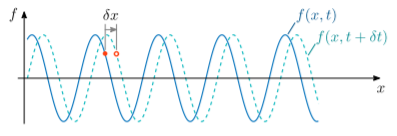# 6.2: Real Solutions to the Wave Equation

$$\newcommand{\vecs}{\overset { \rightharpoonup} {\mathbf{#1}} }$$ $$\newcommand{\vecd}{\overset{-\!-\!\rightharpoonup}{\vphantom{a}\smash {#1}}}$$$$\newcommand{\id}{\mathrm{id}}$$ $$\newcommand{\Span}{\mathrm{span}}$$ $$\newcommand{\kernel}{\mathrm{null}\,}$$ $$\newcommand{\range}{\mathrm{range}\,}$$ $$\newcommand{\RealPart}{\mathrm{Re}}$$ $$\newcommand{\ImaginaryPart}{\mathrm{Im}}$$ $$\newcommand{\Argument}{\mathrm{Arg}}$$ $$\newcommand{\norm}{\| #1 \|}$$ $$\newcommand{\inner}{\langle #1, #2 \rangle}$$ $$\newcommand{\Span}{\mathrm{span}}$$ $$\newcommand{\id}{\mathrm{id}}$$ $$\newcommand{\Span}{\mathrm{span}}$$ $$\newcommand{\kernel}{\mathrm{null}\,}$$ $$\newcommand{\range}{\mathrm{range}\,}$$ $$\newcommand{\RealPart}{\mathrm{Re}}$$ $$\newcommand{\ImaginaryPart}{\mathrm{Im}}$$ $$\newcommand{\Argument}{\mathrm{Arg}}$$ $$\newcommand{\norm}{\| #1 \|}$$ $$\newcommand{\inner}{\langle #1, #2 \rangle}$$ $$\newcommand{\Span}{\mathrm{span}}$$$$\newcommand{\AA}{\unicode[.8,0]{x212B}}$$

We first consider real solutions to the wave equation. One family of solutions are travelling waves of the form $f(x,t) = f_0 \, \cos\!\big(kx - \omega t + \phi\big),\quad\mathrm{where}\;\, \left|\frac{\omega}{k}\right| = v. \label{realsol}$ By direct substitution, we can verify that this satisfies the PDE. We call $$f_0$$ the amplitude of the wave, $$\phi$$ the phase, $$\omega$$ the (angular) frequency, and $$k$$ the wavenumber. By convention, $$\omega$$ is taken to be a positive real number. However, $$k$$ can be either positive or negative, and its sign determines the direction of propagation of the wave; the magnitude of the wavenumber is inversely related to the wavelength $$\lambda$$ by $$\lambda = 2\pi/|k|$$.

As $$t$$ increases, the wave moves to the right if $$k$$ is positive, whereas it moves to the left if $$k$$ is negative. Here’s one way to reason out why this is the case. Consider introducing a small change in time, $$\delta t$$, into the function $$\cos(kx - \omega t + \phi)$$. If, together with this time shift, we change $$x$$ by $$\delta x = (\omega/k)\, \delta t$$, then the change in the $$kx$$ term and the change in the $$\omega t$$ term cancel, leaving the value of the cosine unchanged:Figure $$\PageIndex{1}$$

This implies that the wave shifts by $$\delta x = (\omega/k)\, \delta t$$ during the time interval $$\delta t$$. Hence, the wave velocity is $\textrm{velocity} = \frac{\delta x}{\delta t} = \frac{(\omega/k)\,\delta t}{\delta t} = \frac{\omega}{k}.$ As previously noted, $$\omega$$ is conventionally taken to be a positive real number. Hence, positive $$k$$ implies that the wave is right-moving (positive velocity), and negative $$k$$ implies the wave is left-moving (negative velocity). Moreover, the wave speed is the absolute value of the velocity, which is precisely equal to the constant $$v$$: $\textrm{speed}\; = \, \left|\frac{\delta x}{\delta t}\right| = \frac{\omega}{\left|k\right|} = v.$

## Standing waves

Suppose we have two traveling wave solutions, with equal amplitude and frequency, moving in opposite directions: $f(x,t) = f_0 \, \cos(kx - \omega t + \phi_1) + f_0 \cos(-kx - \omega t + \phi_2).$ Here, we denote $$k = \omega/c$$. Such a superposition is also a solution to the wave equation, called a standing wave. It can be re-written in a variable-separated form (i.e., as the product of a function of $$x$$ and a function of $$t$$): $f(x,t) = 2f_0 \, \cos\big[kx + (\phi_1-\phi_2)/2\big]\, \cos\big[\omega t - (\phi_1+\phi_2)/2\big]. \label{standingsol}$ This can be proven using the trigonometric addition formulas, but the proof is tedious.

This page titled 6.2: Real Solutions to the Wave Equation is shared under a CC BY-SA 4.0 license and was authored, remixed, and/or curated by Y. D. Chong via source content that was edited to the style and standards of the LibreTexts platform; a detailed edit history is available upon request.